# Visualization In Python II : Correlogram, Heat Map, Scatter Graphs

Relation plots are perfectly suited to showing relationships among variables. A scatter plot visualizes the correlation between two variables for one or multiple groups. Bubble plots can be used to show relationships between three variables. The additional third variable is represented by the dot size. Heat maps are great for revealing patterns or correlations between two qualitative variables. A correlogram is a perfect visualization for showing the correlation among multiple variables.

# 1. Scatter Plot

Scatter plots show data points for two numerical variables, displaying a variable on both axes.

• You can detect whether a correlation (relationship) exists between two variables.
• They allow you to plot the relationship between multiple groups or categories using different colors.
• A bubble plot, which is a variation of the scatter plot, is an excellent tool for visualizing the correlation of a third variable.

The following diagram shows a scatter plot of height and weight of persons belonging to a single group: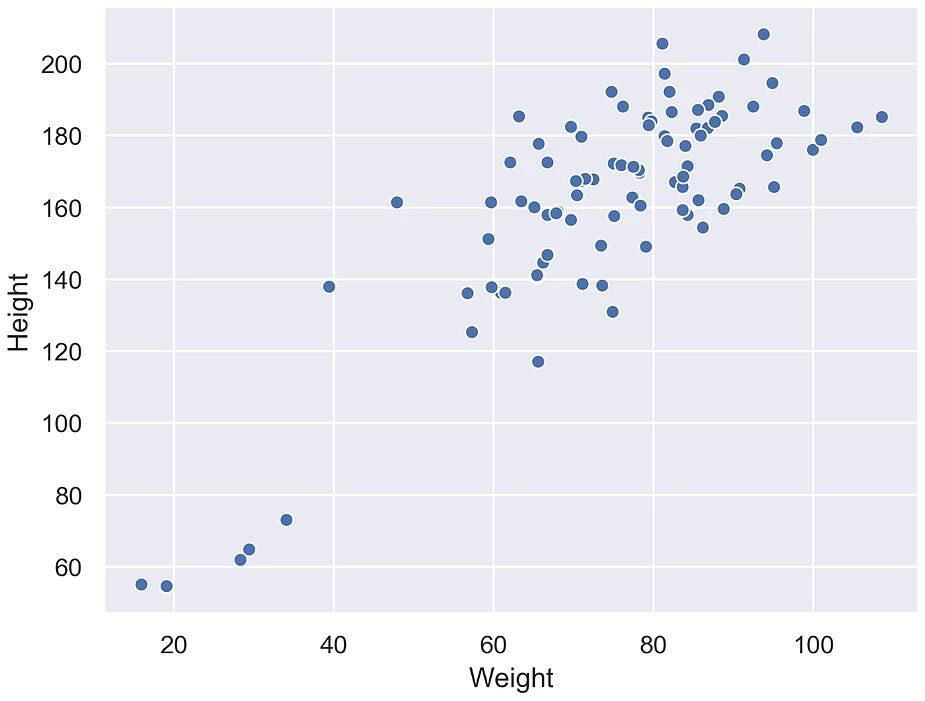Scatter plot of height and weight of persons belonging to a single group

The following diagram shows the same data as in the previous plot but differentiates between groups. In this case, we have different groups: A, B, and C: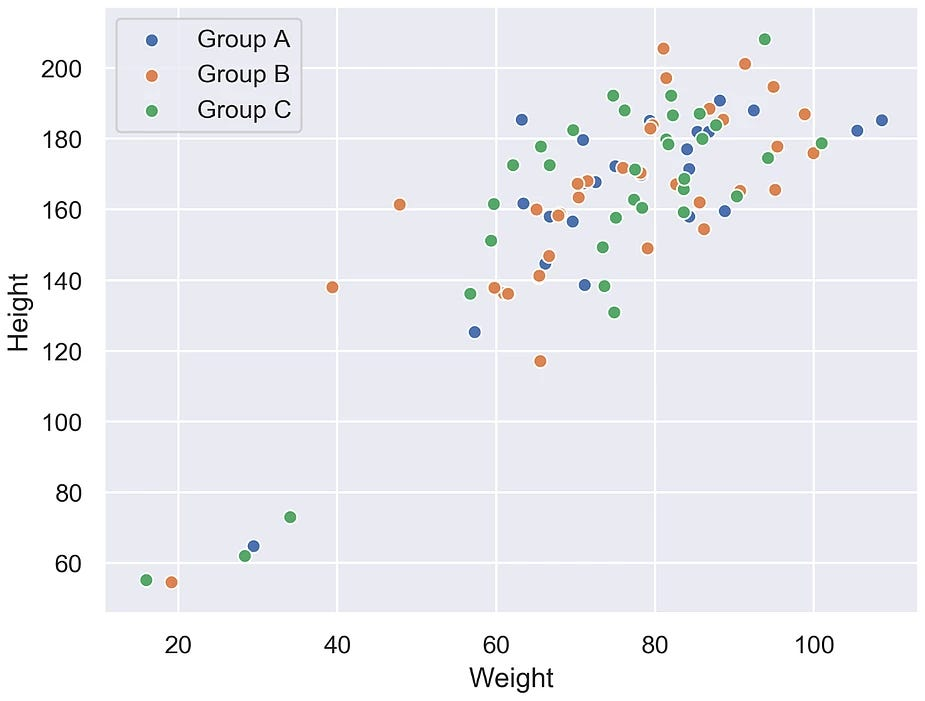Scatter plot of height and weight of persons belonging to a multiple group

The following diagram shows the correlation between body mass and the maximum longevity for various animals grouped by their classes. There is a positive correlation between body mass and maximum longevity: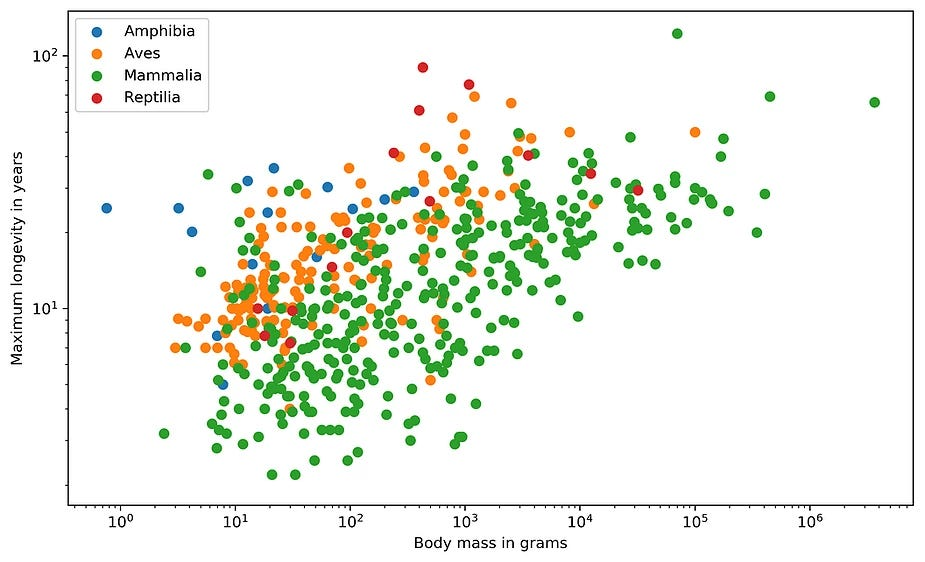Correlation between body mass and the maximum longevity for various animals grouped by their classes

Design Practices

• Start both axes at zero to represent data accurately.
• Use contrasting colors for data points and avoid using symbols for scatter plots with multiple groups or categories.

# 2. Variants: Scatter Plots with Marginal Histograms

In addition to the scatter plot, which visualizes the correlation between two numerical variables, you can plot the marginal distribution for each variable in the form of histograms to give better insight into how each variable is distributed.

The following diagram shows the correlation between body mass and the maximum longevity for animals in the Ave’s class. The marginal histograms are also shown, which helps to get a better insight into both variables: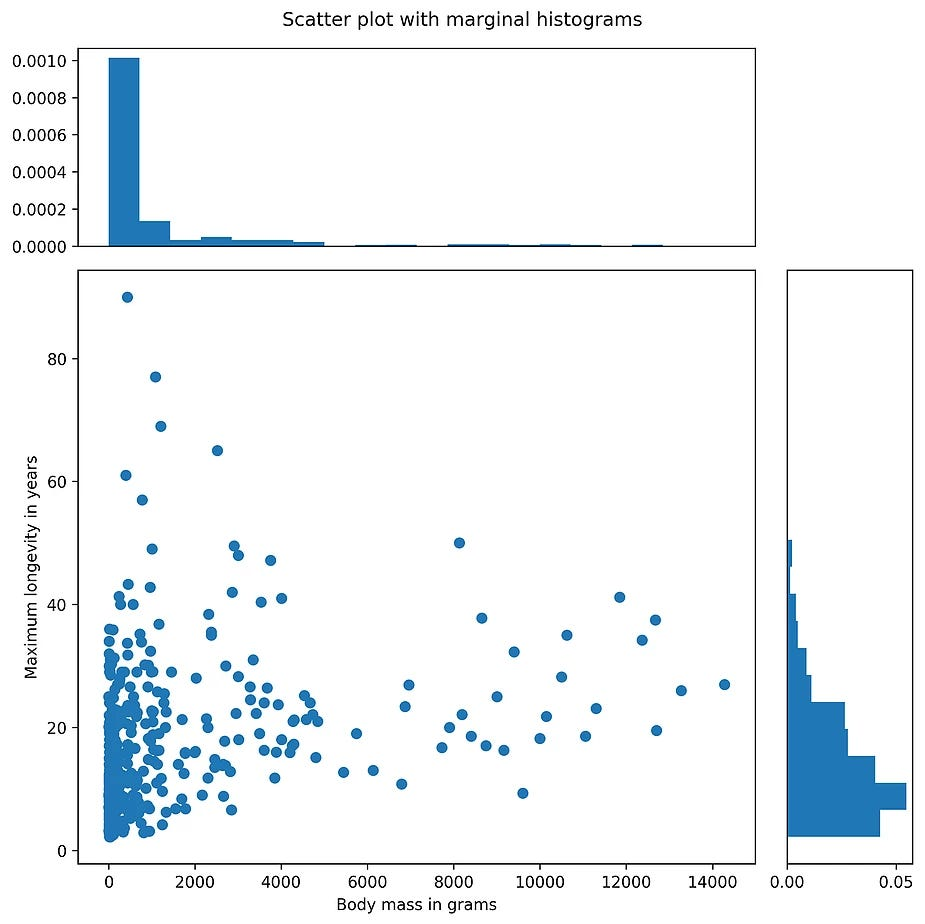Correlation between body mass and the maximum longevity for animals in the Ave’s class

# 3. Bubble Plot

A bubble plot extends a scatter plot by introducing a third numerical variable. The value of the variable is represented by the size of the dots. The area of the dots is proportional to the value. A legend is used to link the size of the dot to an actual numerical value.

Bubble plots help to show a correlation between three variables.

The following diagram shows a bubble plot that highlights the relationship between heights and age of humans to get the weight of each person, which is represented by the size of the bubble: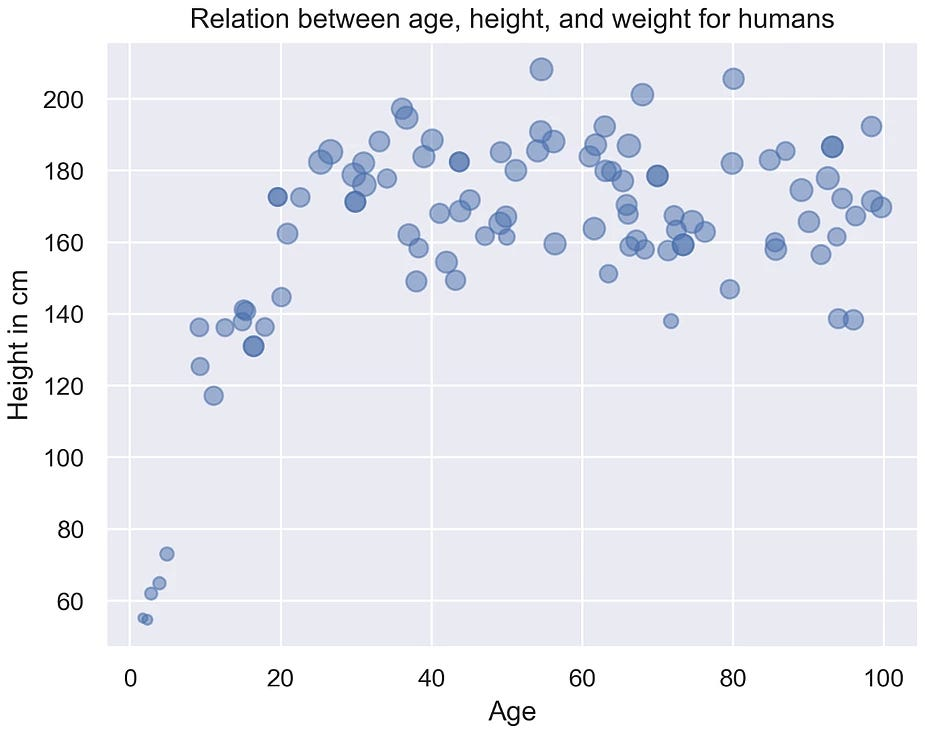bubble plot that highlights the relationship between heights and age of humans

Design Practices

• The design practices for the scatter plot are also applicable to the bubble plot.
• Don’t use bubble plots for very large amounts of data, since too many bubbles make the chart difficult to read.

# 4. Correlogram

A correlogram is a combination of scatter plots and histograms. Histograms will be discussed in detail later in this chapter. A correlogram or correlation matrix visualizes the relationship between each pair of numerical variables using a scatter plot.

The diagonals of the correlation matrix represent the distribution of each variable in the form of a histogram. You can also plot the relationship between multiple groups or categories using different colors. A correlogram is a great chart for exploratory data analysis to get a feel for your data, especially the correlation between variable pairs.

The following diagram shows a correlogram for the height, weight, and age of humans. The diagonal plots show a histogram for each variable. The off-diagonal elements show scatter plots between variable pairs:

The following diagram shows the correlogram with data samples separated by color into different groups:

Design Practices

• Start both axes at zero to represent data accurately.
• Use contrasting colors for data points and avoid using symbols for scatter plots with multiple groups or categories.

# 5. Heatmap

A heat map is a visualization where values contained in a matrix are represented as colors or color saturation. Heat maps are great for visualizing multivariate data (data in which analysis is based on more than two variables per observation), where categorical variables are placed in the rows and columns and a numerical or categorical variable is represented as colors or color saturation.

The visualization of multivariate data can be done using heat maps as they are great for finding patterns in your data.

The following diagram shows a heat map for the most popular products on the electronics category page across various e-commerce websites, where the color shows the number of units sold. In the following diagram, we can analyze that the darker colors represent more units sold, as shown in the key:

Let’s see the same example we saw previously in an annotated heat map, where the color shows the number of units sold:

Design Practice

Select colors and contrasts that will be easily visible to individuals with vision problems so that your plots are more inclusive.

In this article, I introduced various plots for relating a variable to other variables and looked at their uses, and multiple examples for the different relation plots were given.

Check the next post for the part 3 of this articles series.

For more awesome content and regular posts you can connect with me on Instagram😍

--

--

--

## More from Abhijeet Srivastav

CEO Techneophyte | Python Developer | ML Engineer | Data Scientist | Flutter Developer | Penetration Tester | Software Engineer at Infosys

Love podcasts or audiobooks? Learn on the go with our new app.

## Let’s do some machine learning with R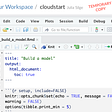## How to predict stock prices with Python + Machine Learning!## CAUSE & EFFECT DIAGRAM(FISH BONE DIAGRAM/ISHIKAWA DIAGRAM)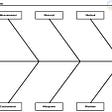## Calling Elections Early: Fake News or Statistics?## Modelling Binary Logistic Regression Using Python (research-oriented modelling and interpretation)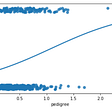## What the census means to maps and the people who build them## Statistics for Data science## Identify customer churn and predict its probability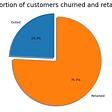## Abhijeet Srivastav

CEO Techneophyte | Python Developer | ML Engineer | Data Scientist | Flutter Developer | Penetration Tester | Software Engineer at Infosys

## Generate Numerical Correlation and Nominal Association Plots using Python## Perform Zonal Statistics on Climate Data with Python## Data Visualisation In Seaborn With Awesome Examples